# COMPSCI 102 Introduction to Discrete Mathematics Graphs II

• Slides: 34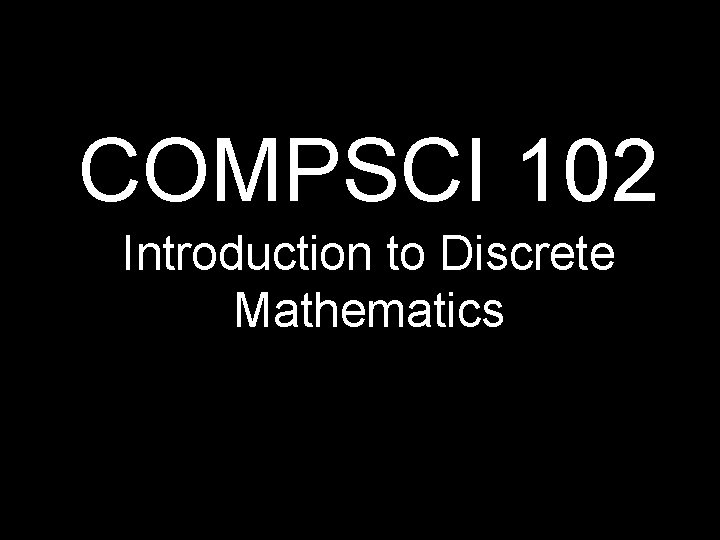COMPSCI 102 Introduction to Discrete Mathematics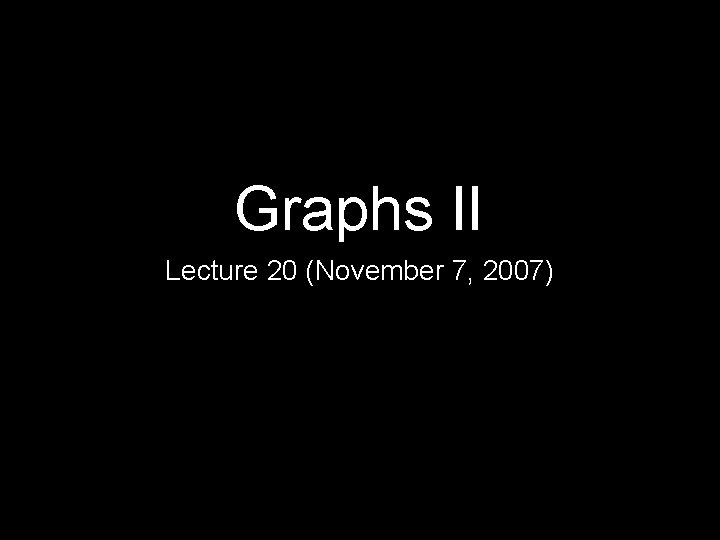Graphs II Lecture 20 (November 7, 2007)RecapTheorem: Let G be a graph with n nodes and e edges The following are equivalent: 1. G is a tree (connected, acyclic) 2. Every two nodes of G are joined by a unique path 3. G is connected and n = e + 1 4. G is acyclic and n = e + 1 5. G is acyclic and if any two non-adjacent points are joined by an edge, the resulting graph has exactly one cycleCayley’s Formula The number of labeled trees on n nodes is nn-2A graph is planar if it can be drawn in the plane without crossing edges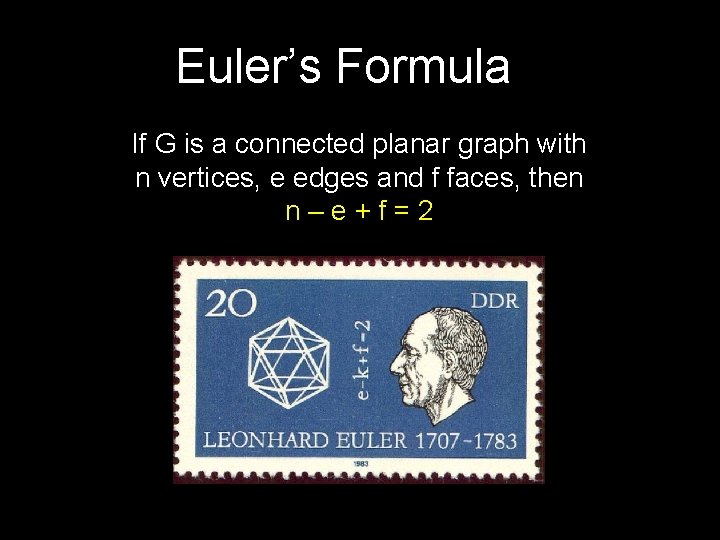Euler’s Formula If G is a connected planar graph with n vertices, e edges and f faces, then n–e+f=2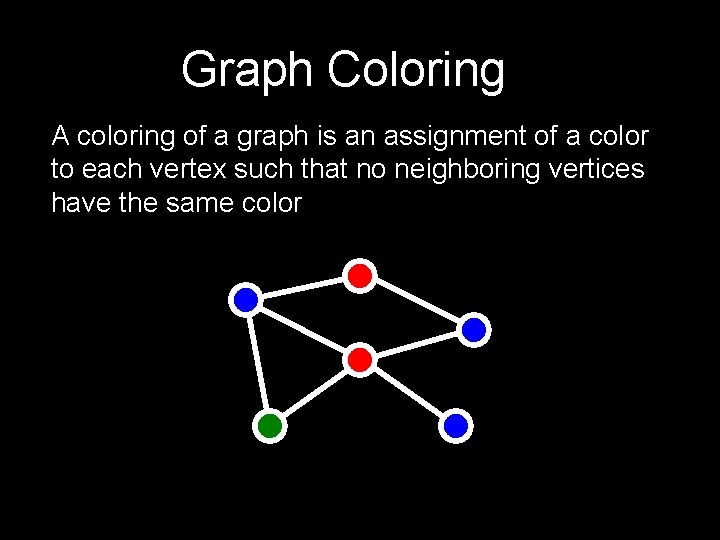Graph Coloring A coloring of a graph is an assignment of a color to each vertex such that no neighboring vertices have the same colorSpanning Trees A spanning tree of a graph G is a tree that touches every node of G and uses only edges from G Every connected graph has a spanning treeFinding Optimal Trees have many nice properties (uniqueness of paths, no cycles, etc. ) We may want to compute the “best” tree approximation to a graph If all we care about is communication, then a tree may be enough. We want a tree with smallest communication link costs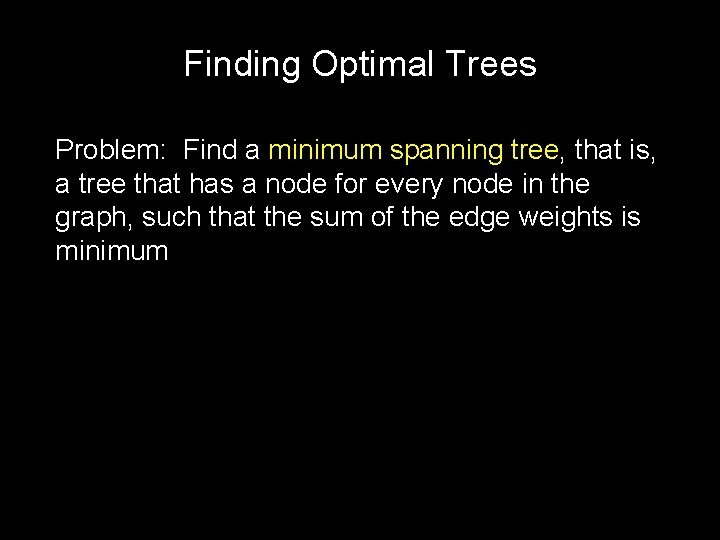Finding Optimal Trees Problem: Find a minimum spanning tree, that is, a tree that has a node for every node in the graph, such that the sum of the edge weights is minimumTree Approximations 7 8 4 5 9 7 8 6 11 9Finding an MST: Kruskal’s Algorithm Create a forest where each node is a separate tree Make a sorted list of edges S While S is non-empty: Remove an edge with minimum weight If it connects two different trees, add the edge. Otherwise discard it.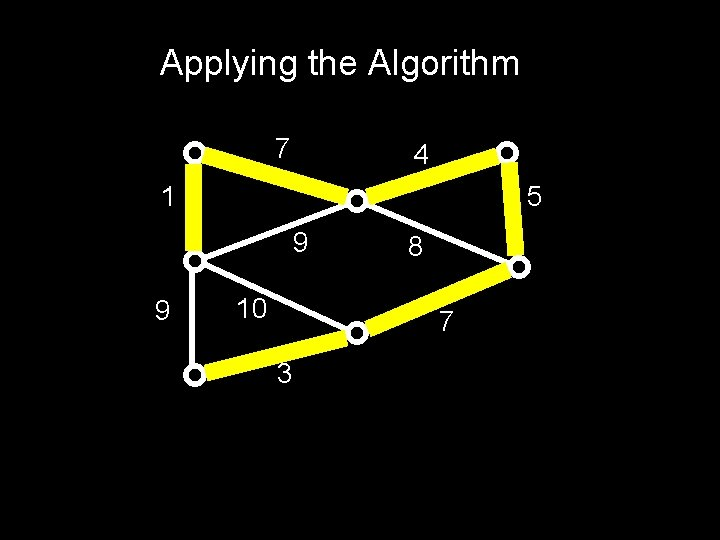Applying the Algorithm 7 4 1 5 9 9 10 8 7 3Analyzing the Algorithm The algorithm outputs a spanning tree T. Suppose that it’s not minimum. (For simplicity, assume all edge weights in graph are distinct) Let M be a minimum spanning tree. Let e be the first edge chosen by the algorithm that is not in M. If we add e to M, it creates a cycle. Since this cycle isn’t fully contained in T, it has an edge f not in T. N = M+e-f is another spanning tree.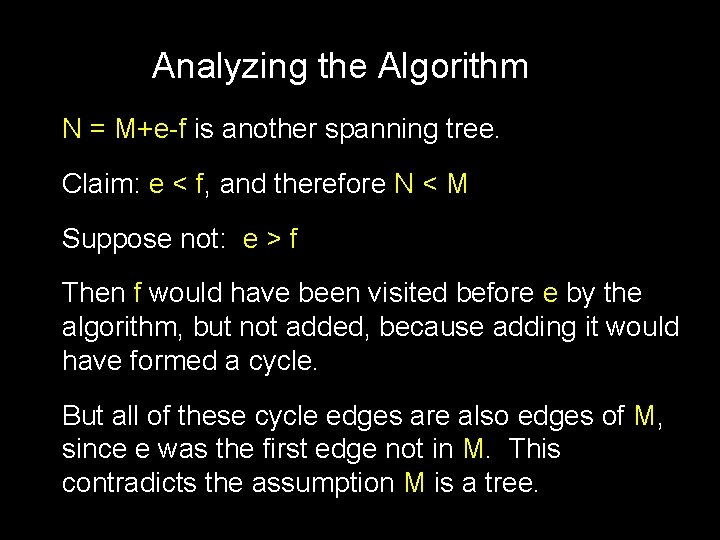Analyzing the Algorithm N = M+e-f is another spanning tree. Claim: e < f, and therefore N < M Suppose not: e > f Then f would have been visited before e by the algorithm, but not added, because adding it would have formed a cycle. But all of these cycle edges are also edges of M, since e was the first edge not in M. This contradicts the assumption M is a tree.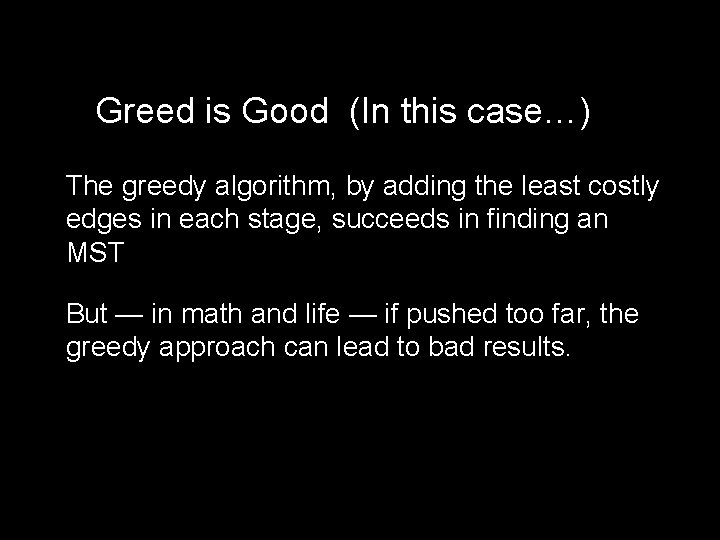Greed is Good (In this case…) The greedy algorithm, by adding the least costly edges in each stage, succeeds in finding an MST But — in math and life — if pushed too far, the greedy approach can lead to bad results.TSP: Traveling Salesman Problem Given a number of cities and the costs of traveling from any city to any other city, what is the cheapest round-trip route that visits each city exactly once and then returns to the starting city?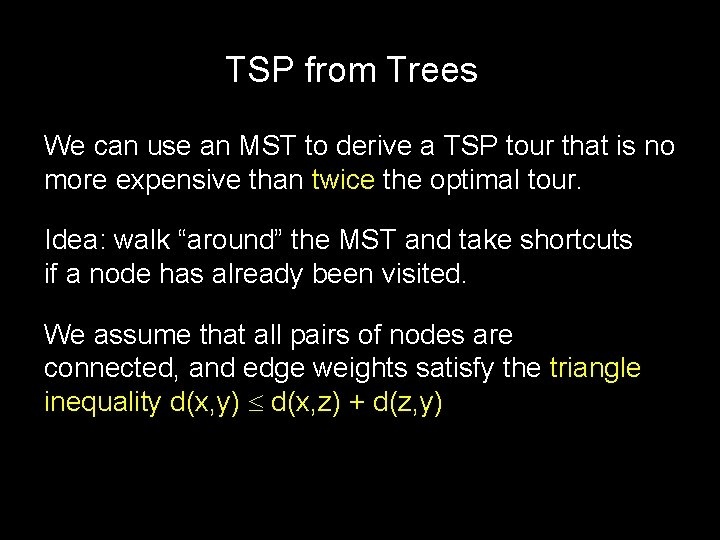TSP from Trees We can use an MST to derive a TSP tour that is no more expensive than twice the optimal tour. Idea: walk “around” the MST and take shortcuts if a node has already been visited. We assume that all pairs of nodes are connected, and edge weights satisfy the triangle inequality d(x, y) d(x, z) + d(z, y)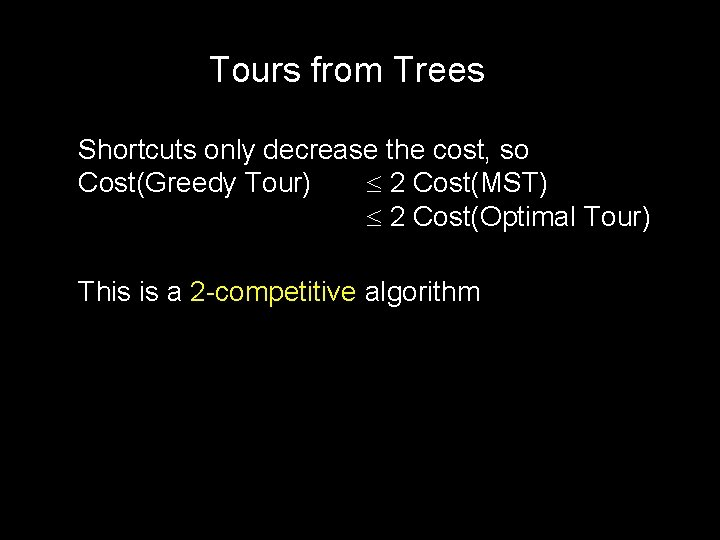Tours from Trees Shortcuts only decrease the cost, so Cost(Greedy Tour) 2 Cost(MST) 2 Cost(Optimal Tour) This is a 2 -competitive algorithm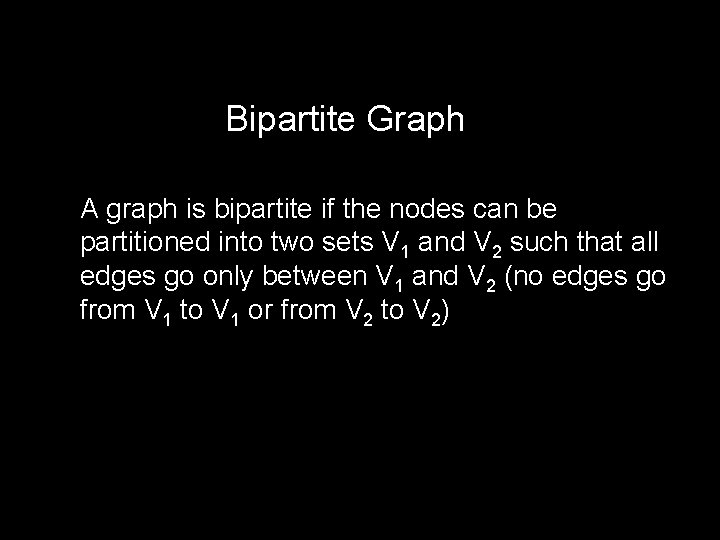Bipartite Graph A graph is bipartite if the nodes can be partitioned into two sets V 1 and V 2 such that all edges go only between V 1 and V 2 (no edges go from V 1 to V 1 or from V 2 to V 2)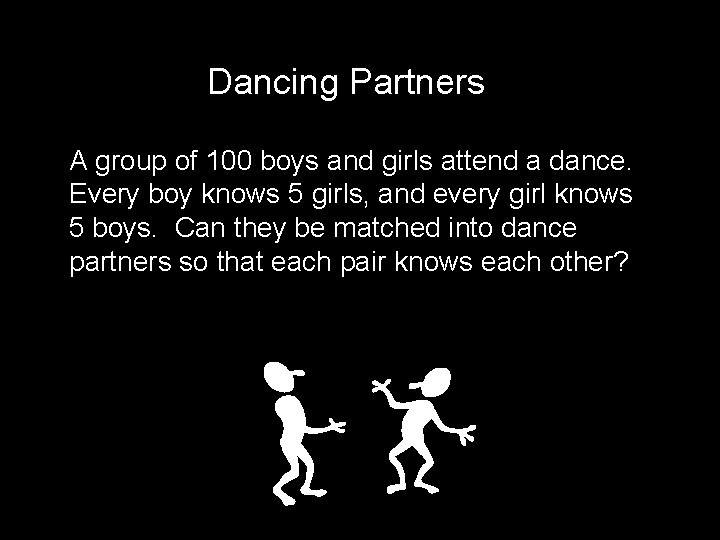Dancing Partners A group of 100 boys and girls attend a dance. Every boy knows 5 girls, and every girl knows 5 boys. Can they be matched into dance partners so that each pair knows each other?Dancing PartnersPerfect Matchings Theorem: If every node in a bipartite graph has the same degree d 1, then the graph has a perfect matching. Note: if degrees are the same then |A| = |B|, where A is the set of nodes “on the left” and B is the set of nodes “on the right”A Matter of Degree Claim: If degrees are the same then |A| = |B| Proof: If there are m boys, there are md edges If there are n girls, there are nd edges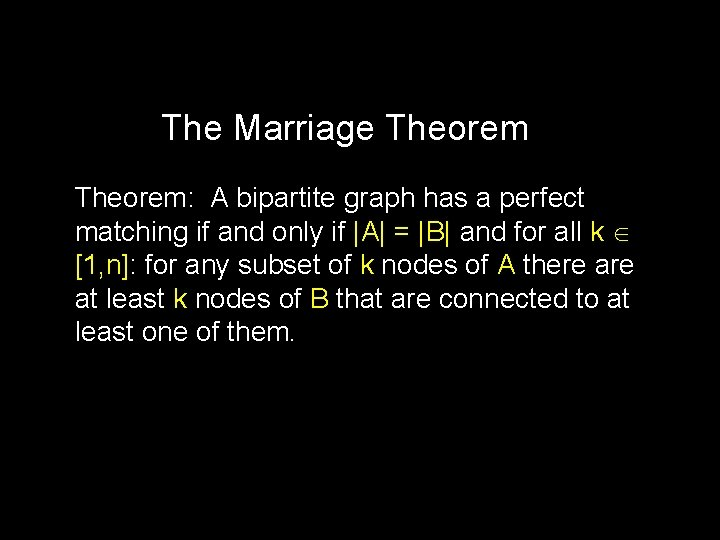The Marriage Theorem: A bipartite graph has a perfect matching if and only if |A| = |B| and for all k [1, n]: for any subset of k nodes of A there at least k nodes of B that are connected to at least one of them.The Marriage Theorem For any subset of (say) k nodes of A there at least k nodes of B that are connected to at least one of them The condition fails for this graphThe Feeling is Mutual At least k At most n-k k n-k The condition of theorem still holds if we swap the roles of A and B: If we pick any k nodes in B, they are connected to at least k nodes in AProof of Marriage Theorem Call a bipartite graph “matchable” if it has the same number of nodes on left and right, and any k nodes on the left are connected to at least k on the right Strategy: Break up the graph into two matchable parts, and recursively partition each of these into two matchable parts, etc. , until each part has only two nodes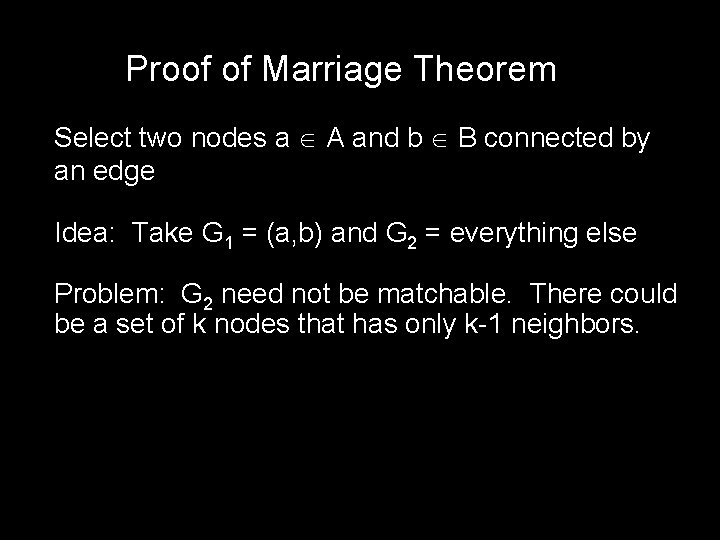Proof of Marriage Theorem Select two nodes a A and b B connected by an edge Idea: Take G 1 = (a, b) and G 2 = everything else Problem: G 2 need not be matchable. There could be a set of k nodes that has only k-1 neighbors.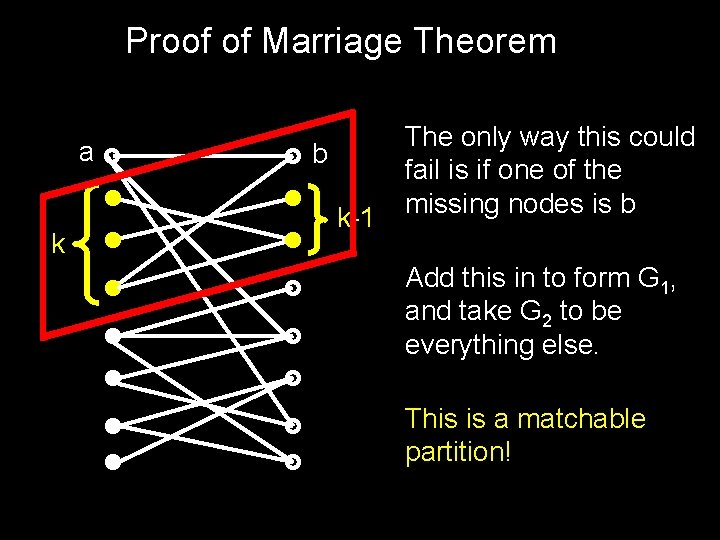Proof of Marriage Theorem a k b k-1 The only way this could fail is if one of the missing nodes is b Add this in to form G 1, and take G 2 to be everything else. This is a matchable partition!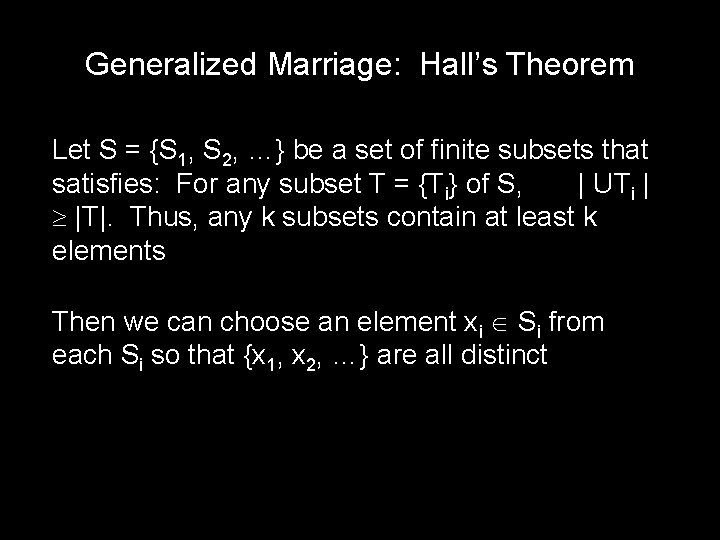Generalized Marriage: Hall’s Theorem Let S = {S 1, S 2, …} be a set of finite subsets that satisfies: For any subset T = {Ti} of S, | UTi | |T|. Thus, any k subsets contain at least k elements Then we can choose an element xi Si from each Si so that {x 1, x 2, …} are all distinctExample Suppose that a standard deck of cards is dealt into 13 piles of 4 cards each Then it is possible to select a card from each pile so that the 13 chosen cards contain exactly one card of each rank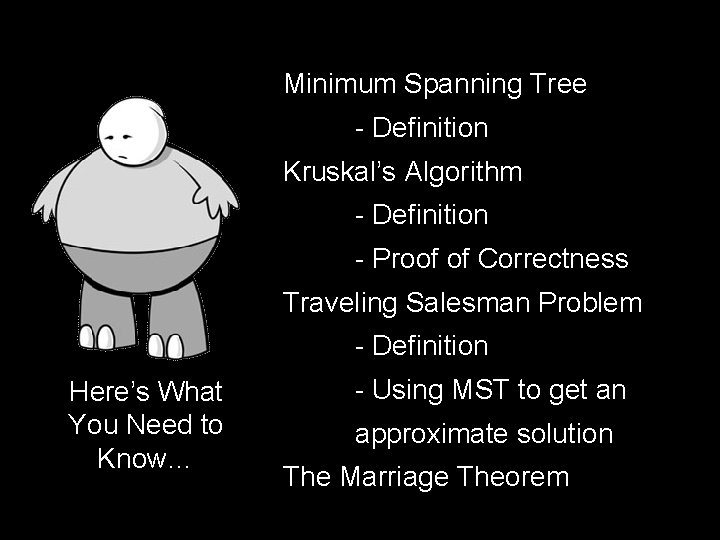Minimum Spanning Tree - Definition Kruskal’s Algorithm - Definition - Proof of Correctness Traveling Salesman Problem - Definition Here’s What You Need to Know… - Using MST to get an approximate solution The Marriage Theorem